• Index
•
•

## Comparison with Analytical Bearing Capacity

In this example, the analytical solution for undrained bearing capacity given by Prandtl from plasticity theory will be compared with the limit equilibrium result calculated using MacSlope, using Spencer's method of slices with both circular and non-circular slip surfaces.

We consider the problem below in the undrained condition (φ = 0), with a surcharge q equal to 100 kPa acting on a weightless soil having an undrained cohesion cu of 20 kPa. The surcharge is applied over a width B = 5m.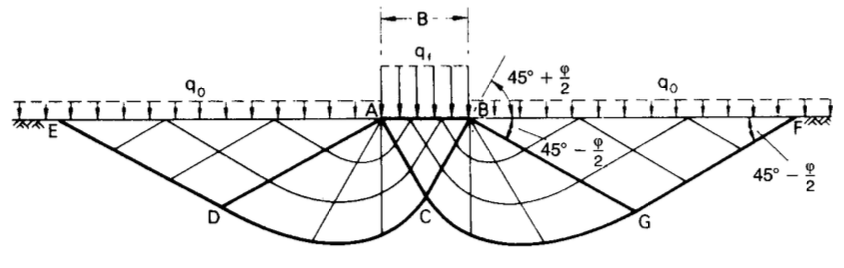The ultimate bearing capacity for the undrained case, given by plasticity theory, is: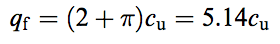which is equal to 5.14*(20 kPa) = 102.8 kPa for this case. We calculate the theoretical factor of safety (FOS) as: qu / q = 102.8 kPa / 100 kPa = 1.028.

## Circular Slip Surface

To locate the critical circular slip surface for the problem, we conduct an Auto Search. The factor of safety is calculated as 1.103 and the critical circular slip surface is shown below. Note that the critical slip circle center is positioned in the middle of the slip surface.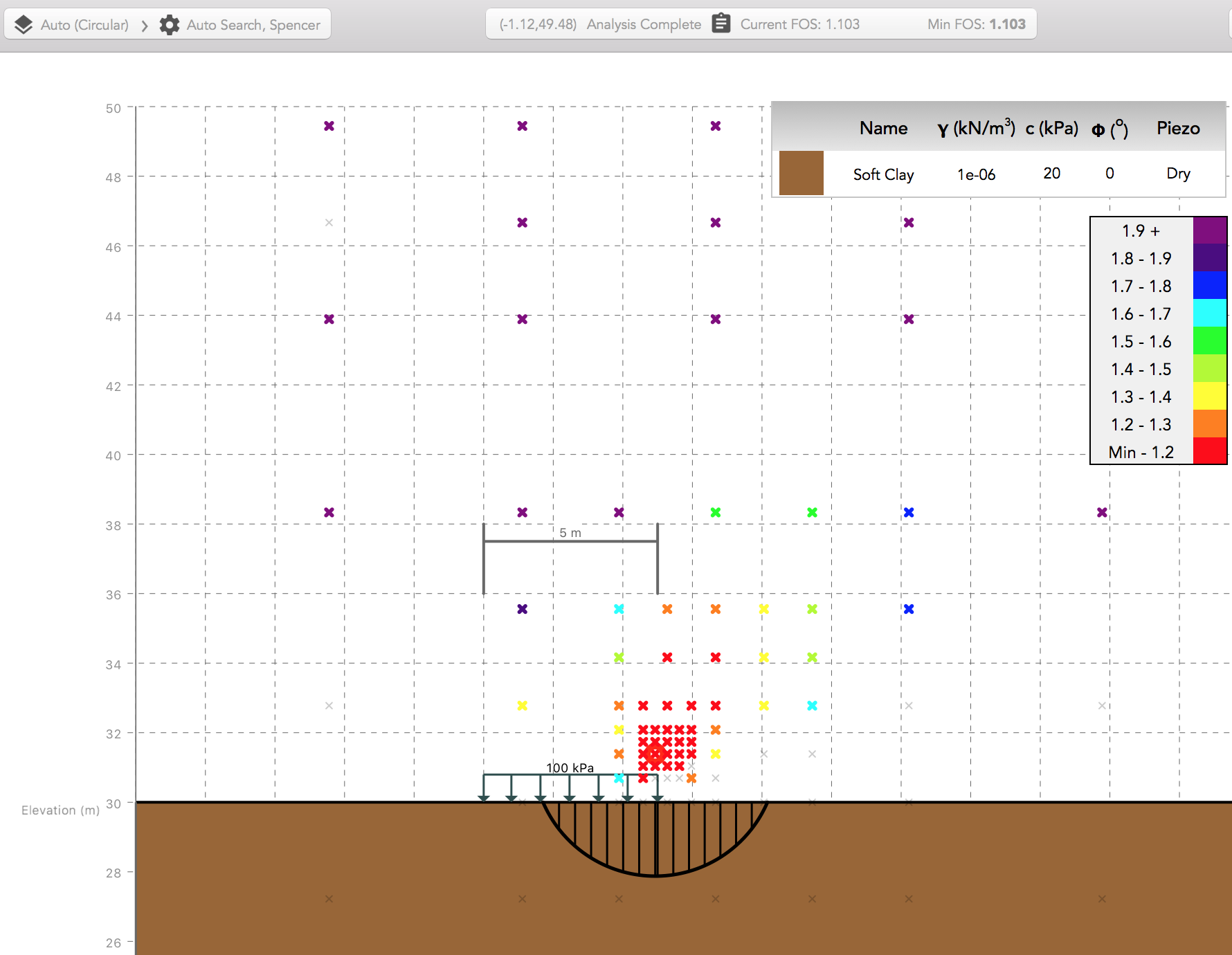To verify this result, we consider the moment equilbrium of a circular slip surface rotating about a point O as shown below.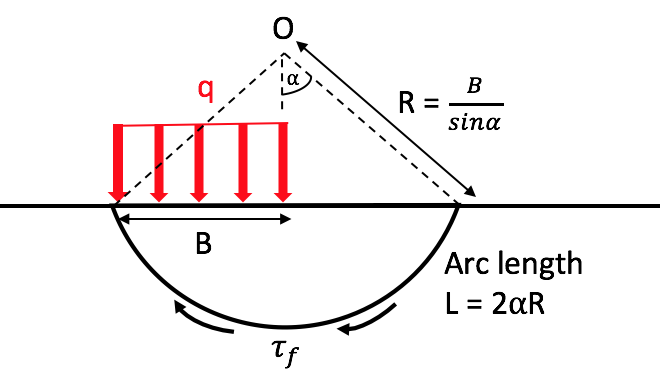At failure (FOS = 1), we have: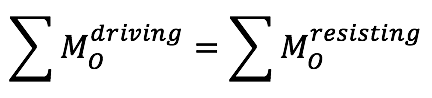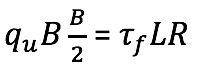Substituting the above trigonometric relations for L and R and solving for the bearing capacity (qu), we get: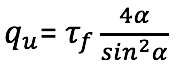By deriving the bearing capacity with respect to the angle α, we solve for the angle α that gives the minimum bearing capacity qu, and therefore the minimum FOS for this problem.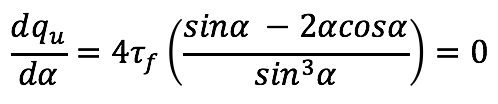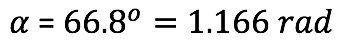Substituting this angle α into the bearing capacity equation along with the undrained shear strength of 20 kPa gives us a bearing capacity of 110.4 kPa, or a factor of safety FOS = qu / q = 110.4 kPa / 100 kPa = 1.104, a value nearly identical to that calculated using the Auto Search.

In this case, the circular slip surface failure mechanism overestimates the factor of safety compared to the analytical solution. Although we have successfully located the minimum circular slip surface, it approaches the true solution from the unsafe side.

## Non-Circular Slip Surface

To locate the critical non-circular slip surface for the problem, we begin with the critical circular trial slip surface calculated from the auto search, and then attempt the Optimization procedure on the trial. The factor of safety is calculated as 0.806 and the slip surface is shown below.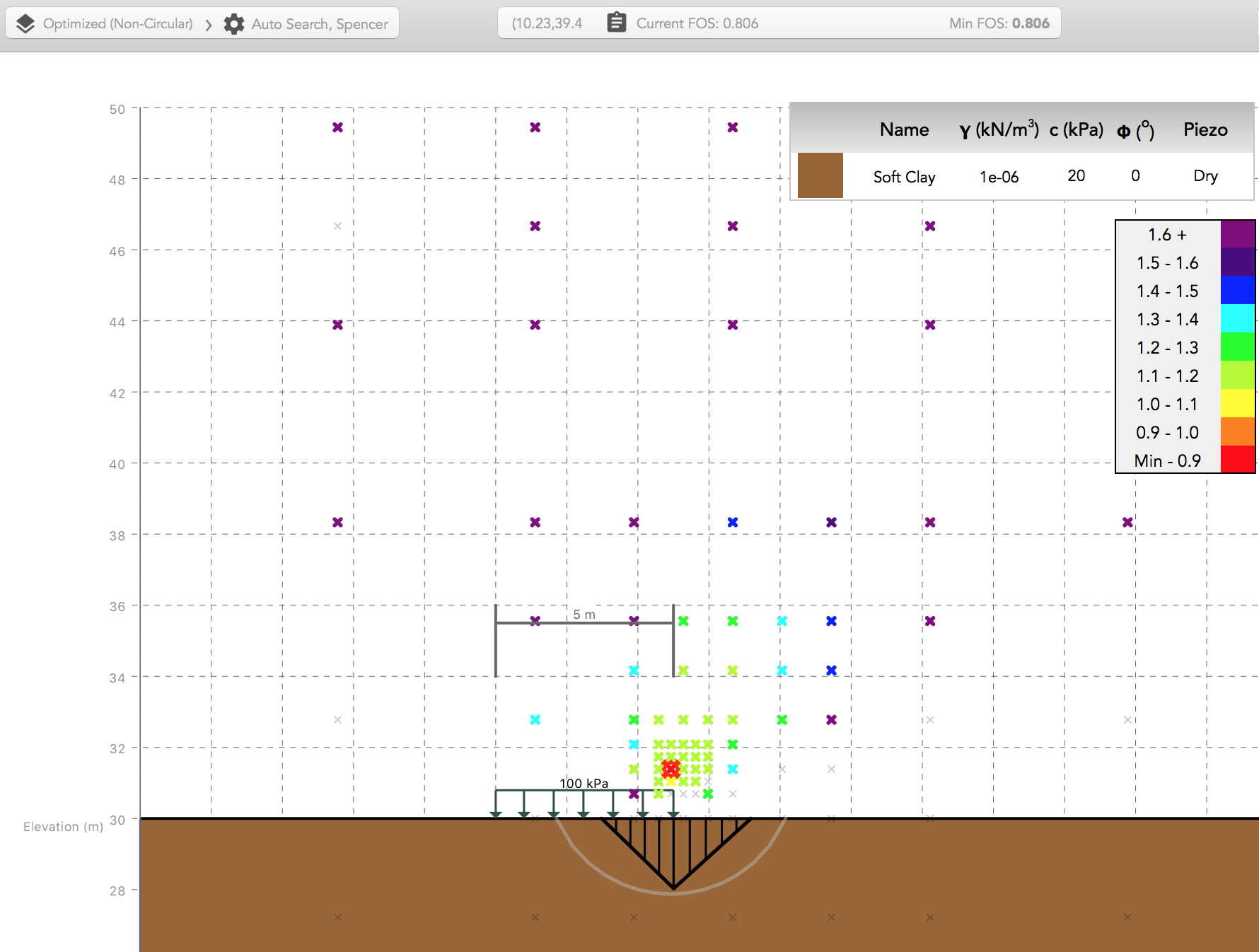In this case, the optimized non-circular slip surface failure mechanism underestimates the factor of safety compared to the analytical solution. Although the optimization procedure has located this statically correct slip surface, observing the shape of the surface, we note that it is not realistic, as the sharp kink present is not kinematically possible in typical uniform, isotropic soils.

## Comparison with Kinematically Admissible Surface

Despite the fact that the limit equilibrium methods used do not consider the plastic behaviour of soil, we should expect a similar result if the postulated slip surface is equivalent to that given by the plasticity theory. To compare, we construct a specified surface of the Prandtl bearing capacity surface. In this undrained case, it consists of two wedges inclined at 45o, joined by a circular fan.

The factor of safety is calculated as 0.965, a value comparable to the theoretical 1.028 for this example, and the slip surface is shown below.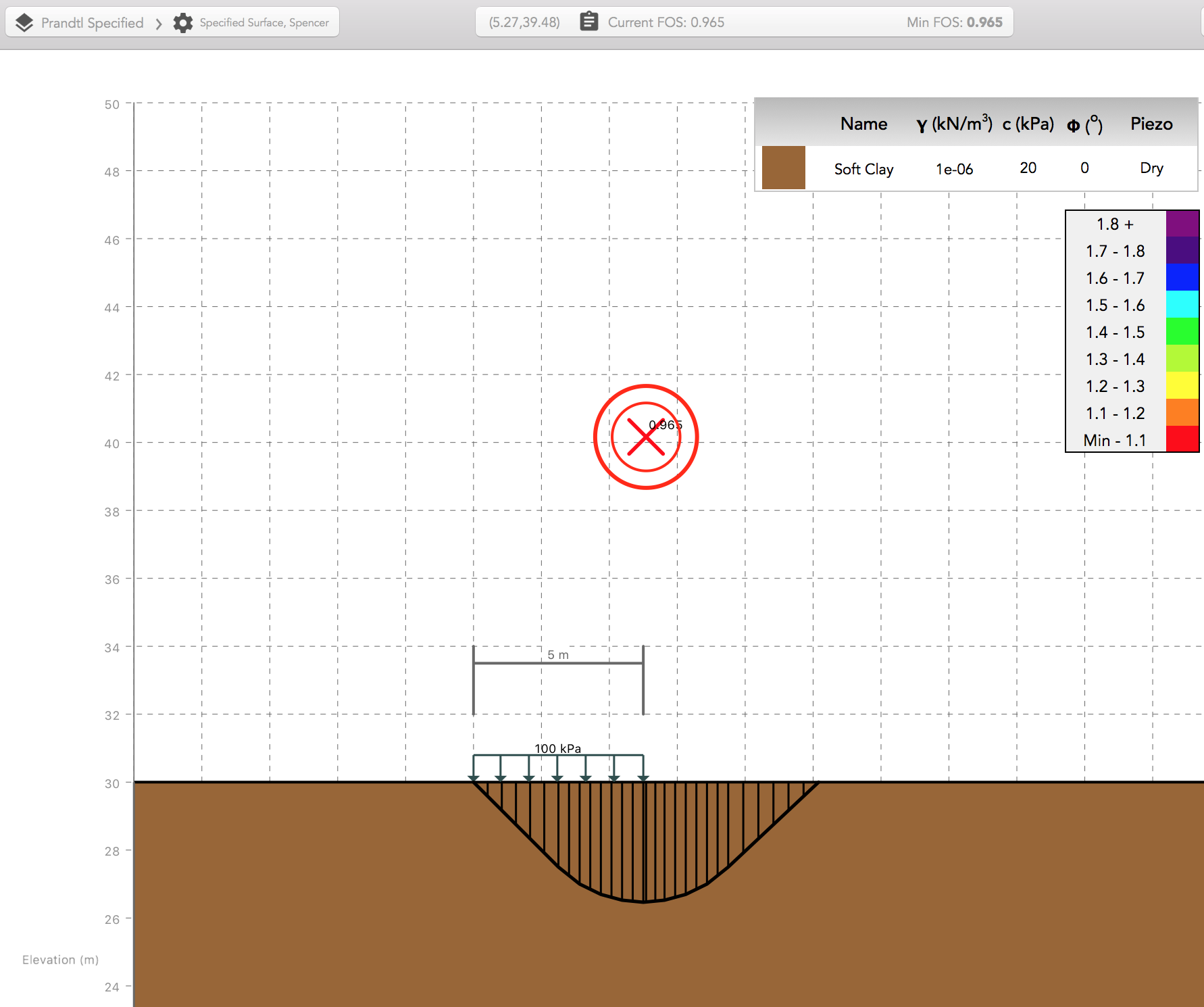## Summary

While the results for the circular and non-circular slip surfaces provide a useful upper and lower bound to the analytical solution, they highlight the need to interpret limit equilibrium calculations with care. Although both the circular and optimized non-circular soil masses are in static force and moment equilibrium, neither gives us an adequate solution. The circular slip surface, while kinematically possible, does not represent the critical surface from plasticity theory, and the optimized non-circular surface is not physically possible.
The results are not surprising because the limit equilibrium methods used in MacSlope do not consider the work required to plastically deform the soil according to the postulated slip surfaces. However, it has been shown that limit equilibrium analysis can provide accurate solutions to many slope stability problems when the shape of the slip surface is realistic, and when we use the analytical bearing capacity slip surface in the limit equilibrium analysis, the result is reasonable.

When assessing whether slip surfaces postulated by MacSlope are representative and feasible, it is helpful to question:

- Could this circular slip surface be optimized?
Although circular slip surfaces can provide an excellent approximation to many observed slope failure shapes, in many cases the critical slip surface shape is non-circular, due to anisotropy, discontinuities, loading conditions, or to satisfy kinematic equilibrium such as in this bearing capacity problem. In most cases it is recommended to attempt optimization of the critical circular slip surface to locate a feasible slip surface with a lower FOS. In other cases it may be appropriate to model composite slip surfaces using Impenetrable materials so that part of the slip surface remains circular.

- Is the slip surface physically possible?
Circular slip surfaces are generally physically admissible, being of a rotational nature, they provide suitable active and passive wedges. If, however, after optimizing a circular slip surface it takes a shape which is clearly kinematically inadmissible, such as in this bearing capacity example, it should not be considered correct. The Force Concave option for the optimization procedure can be used to correct convex slip surfaces, although sometimes the critical slip surface may include a convex portion due to some anisotropy.

Download the MacSlope file with the analysis results for this example here.

### References

• Greco, V. R. 1996 . “Efficient Monte Carlo technique for locating critical slip surface.” J. Geotech. Engrg., 122 7 , 517–525.
• Yu, H.S., R. Salgado, S.W. Sloane and J.M. Kim. (1999) ‘Limit Analysis versus Limit Equilibrium for Slope Stability.’ ASCE Journal of Geotechnical and Geoenvironmental Engineering, ASCE, 125(10), 915-918.
• University of the West of England (2001), Bearing Capacity, http://environment.uwe.ac.uk/geocal/foundations/founbear.htm